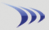2-period RSI - Scan to find declining RSI Rate this Topic:Previous Topic · Next Topic Watch this topic · Print this topic ·
kenoracle
 Posted : Tuesday, May 20, 2014 7:47:50 PM
Registered User
Joined: 1/13/2012
Posts: 72

I am looking at RSI, 2-period.  I need the RSI to be declining for 4 days in a row..

At the 4th day, RSI needs to be < 10.  All days (1 - 4) must be declining.

Ken.

kenoracle
 Posted : Tuesday, May 20, 2014 8:01:10 PM
Registered User
Joined: 1/13/2012
Posts: 72

Answer found!  No need for work on your part.  Here is the request in context...

RSI2.1 < RSI2.1.1 < RSI2.1.2 < RSI2.1.3 < RSI2.1.4 AND
C > AVGC200 AND
RSI2.1 < 10 AND
RSI2.4 < 60

Thanks again.

Bruce_L
 Posted : Wednesday, May 21, 2014 3:15:10 PMWorden Trainer

Joined: 10/7/2004
Posts: 65,138

There are actually two possibilities as to the settings of a 2-period Wilder's RSI. The RSI in the formula you have written does not use Wilder's smoothing. In this instance, you would need to modify your formula slightly to actually make all of the desired comparisons as each of these comparisons needs to be made individually instead of in series.

RSI2 < 10 AND RSI2 < RSI2.1.1 AND RSI2.1.1 < RSI2.1.2 AND RSI2.1.2 < RSI2.1.3 AND RSI2.1.3 < RSI2.1.4 AND RSI2.1.4 < 60 AND AVGC200 < C

The formula for a 2-period Wilder's RSI with Wilder's smoothing is quite a bit longer than just RSI2.

50 * (C - XAVGC3.1) / 2 / (.5000305 * (ABS(C - C1) + .5 * (ABS(C1 - C2) + .5 * (ABS(C2 - C3) + .5 * (ABS(C3 - C4) + .5 * (ABS(C4 - C5) + .5 * (ABS(C5 - C6) + .5 * (ABS(C6 - C7) + .5 * (ABS(C7 - C8) + .5 * (ABS(C8 - C9) + .5 * (ABS(C9 - C10) + .5 * (ABS(C10 - C11) + .5 * (ABS(C11 - C12) + .5 * (ABS(C12 - C13) + .5 * (ABS(C13 - C14)))))))))))))))) + 50

But a 2-period Wilder's RSI with Wilder's smoothing should be less than its previous value if and only if price is also less than its previous value. So we can check for the current value to be less than 10, the value of 4 bars ago to be less than 60 and then just compare closing prices for the parts in the middle.

50 * (C - XAVGC3.1) / 2 / (.5000305 * (ABS(C - C1) + .5 * (ABS(C1 - C2) + .5 * (ABS(C2 - C3) + .5 * (ABS(C3 - C4) + .5 * (ABS(C4 - C5) + .5 * (ABS(C5 - C6) + .5 * (ABS(C6 - C7) + .5 * (ABS(C7 - C8) + .5 * (ABS(C8 - C9) + .5 * (ABS(C9 - C10) + .5 * (ABS(C10 - C11) + .5 * (ABS(C11 - C12) + .5 * (ABS(C12 - C13) + .5 * (ABS(C13 - C14)))))))))))))))) + 50 < 10 AND C < C1 AND C1 < C2 AND C2 < C3 AND C3 < C4 AND 50 * (C4 - XAVGC3.5) / 2 / (.5000305 * (ABS(C4 - C5) + 1 / 2 * (ABS(C5 - C6) + 1 / 2 * (ABS(C6 - C7) + 1 / 2 * (ABS(C7 - C8) + 1 / 2 * (ABS(C8 - C9) + 1 / 2 * (ABS(C9 - C10) + 1 / 2 * (ABS(C10 - C11) + 1 / 2 * (ABS(C11 - C12) + 1 / 2 * (ABS(C12 - C13) + 1 / 2 * (ABS(C13 - C14) + 1 / 2 * (ABS(C14 - C15) + 1 / 2 * (ABS(C15 - C16) + 1 / 2 * (ABS(C16 - C17) + 1 / 2 * (ABS(C17 - C18)))))))))))))))) + 50 < 60 AND AVGC200 < C

-Bruce
Personal Criteria Formulas
TC2000 Support Articles
kenoracle
 Posted : Wednesday, May 21, 2014 3:22:09 PM
Registered User
Joined: 1/13/2012
Posts: 72

Thanks for the alternatives, Bruce.  I will try them.

Thanks.

Ken.

ptaglia1
 Posted : Thursday, January 24, 2019 11:25:48 AMGold Customer

Joined: 10/7/2004
Posts: 9

Hi Bruce,

Is it possible to work with you privately and to pay you an hourly fee to help construct alogorithms for me?

If yes, please email me at p_taglia@yahoo.com

Thank you!

Bruce_L
 Posted : Thursday, January 24, 2019 11:42:18 AMWorden Trainer

Joined: 10/7/2004
Posts: 65,138

You can schedule a personal training session with technical support. The cost is \$95 / hour in minimum increments of an hour.

-Bruce
Personal Criteria Formulas
TC2000 Support Articles
Users browsing this topic
Guest-1

 Forum Jump Customer Training & Support - Ask a Trainer - TC2000 version 12/18 - Ask a Trainer - TC2000 version 7 - Ask a Trainer - StockFinder 5.0 - PCFs, EasyScan and Custom Indicators General Discussions - Stock and Market Talk - TC2000 version 12 or 18 - TC2000 version 7 - StockFinder 5.0 - RealCode for StockFinder 5.0 Tutorial Videos - TC2000 version 12 tutorial videos - TC2000 version 7 tutorial videos You cannot post new topics in this forum. You cannot reply to topics in this forum. You cannot delete your posts in this forum. You cannot edit your posts in this forum. You cannot create polls in this forum. You cannot vote in polls in this forum.